Confidence Interval

The QUANTREG procedure provides three methods to compute confidence intervals for the regression quantile parameter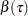: sparsity, rank, and resampling. The sparsity method is the most direct and the fastest, but it involves estimation of the sparsity function, which is not robust for data that are not independently and identically distributed. To deal with this problem, the QUANTREG procedure computes a Huber sandwich estimate by using a local estimate of the sparsity function. The rank method, which computes confidence intervals by inverting the rank score test, does not suffer from this problem, but it uses the simplex algorithm and is computationally expensive with large data sets. The resampling method, which uses the bootstrap approach, addresses these problems, but at a computation cost.

Based on these properties, the QUANTREG uses a combination of the resampling and rank methods as the default. For data sets with more than either 5,000 observations or 20 variables, the QUANTREG procedure uses the MCMB resampling method; otherwise it uses the rank method. You can request a particular method by using the CI= option in the PROC statement.

Sparsity

Consider the linear model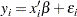and assume that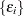,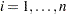, are iid with a distribution F and a density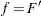, where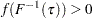in a neighborhood of. Under some mild conditions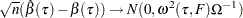where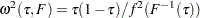and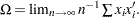Refer to Koenker and Bassett (1982).

This asymptotic distribution for the regression quantile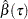can be used to construct confidence intervals. However, the reciprocal of the density function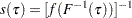which is called the sparsity function, must first be estimated.

Since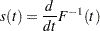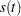can be estimated by the difference quotient of the empirical quantile function—that is,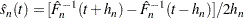where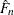is an estimate of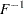and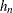is a bandwidth that tends to zero as.

The QUANTREG procedure provides two bandwidth methods. The Bofinger bandwidth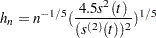is an optimizer of mean squared error for standard density estimation, and the Hall-Sheather bandwidth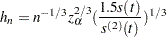is based on Edgeworth expansions for studentized quantiles, where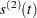is the second derivative ofand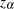satisfies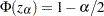for the construction of
confidence intervals. The quantity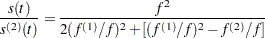is not sensitive to f and can be estimated by assuming f is Gaussian.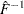can be estimated by the empirical quantile function of the residuals from the quantile regression fit,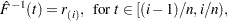or the empirical quantile function of regression proposed by Bassett and Koenker (1982),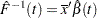The QUANTREG procedure interpolates the first empirical quantile function and gets the piecewise linear version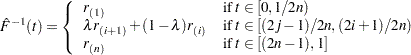is set to a constant if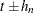falls outside
.

This estimator of the sparsity function is sensitive to the iid assumption. Alternately, Koenker and Machado (1999) considered the non-iid case. By assuming local linearity of the conditional quantile function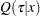in x, they proposed a local estimator of the density function by using the difference quotient. A Huber sandwich estimate of the covariance and standard error is computed and used to construct the confidence intervals. One difficulty with this method is the selection of the bandwidth when using the difference quotient. With a small sample size, either the Bofinger or the Hall-Sheather bandwidth tends to be too large to assure local linearity of the conditional quantile function. The QUANTREG procedure uses a heuristic bandwidth selection in these cases.

By default, the QUANTREG procedure computes non-iid confidence intervals. You can request iid confidence intervals with the IID option in the PROC statement.

Inversion of Rank Tests

The classical theory of rank tests can be extended to test the hypothesis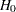: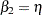in the linear regression model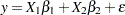. Here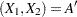. See Gutenbrunner and Jureckova (1992) for more details. By inverting this test, confidence intervals can be computed for the regression quantiles that correspond to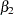.

The rank score function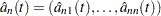can be obtained by solving the dual problem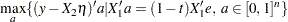For a fixed quantile, integrating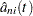with respect to the-quantile score function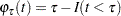yields the-quantile scores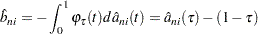Under the null hypothesis: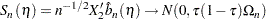for large n, where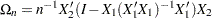.

Let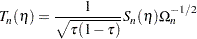Then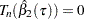from the constraint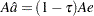in the full model. In order to obtain confidence intervals for, a critical value can be specified for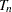. The dual vector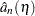is a piecewise constant in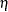, andcan be altered without compromising the optimality ofas long as the signs of the residuals in the primal quantile regression problem do not change. Whengets to such a boundary, the solution does change, but can be restored by taking one simplex pivot. The process can continue in this way until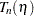exceeds the specified critical value. Sinceis piecewise constant, interpolation can be used to obtain the desired level of confidence interval; see Koenker and d’Orey (1994).

Resampling

The bootstrap can be implemented to compute confidence intervals for regression quantile estimates. As in other regression applications, both the residual bootstrap and the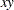-pair bootstrap can be used. The former assumes iid random errors and resamples from the residuals, while the later resamplespairs and accommodates some forms of heteroscedasticity. Koenker (1994) considered a more interesting resampling mechanism, resampling directly from the full regression quantile process, which he called the Heqf bootstrap.

In contrast with these bootstrap methods, Parzen, Wei, and Ying (1994) observed that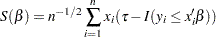which is the estimating equation for theth regression quantile, is a pivotal quantity for theth quantile regression parameter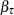. In other words, the distribution of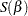can be generated exactly by a random vector
, which is a weighted sum of independent, re-centered Bernoulli variables. They further showed that for large n, the distribution of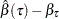can be approximated by the conditional distribution of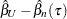, where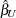solves an augmented quantile regression problem with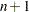observations with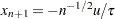and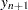sufficiently large for a given realization of u. By exploiting the asymptotically pivot role of the quantile regression "gradient condition," this approach also achieves some robustness to certain heteroscedasticity.

Although the bootstrap method by Parzen, Wei, and Ying (1994) is much simpler, it is too time-consuming for relatively large data sets, especially for high-dimensional data sets. The QUANTREG procedure implements a new, general resampling method developed by He and Hu (2002), which is referred to as the Markov chain marginal bootstrap (MCMB). For quantile regression, the MCMB method has the advantage that it solves p one-dimensional equations instead of p-dimensional equations, as do the previous bootstrap methods. This greatly improves the feasibility of the resampling method in computing confidence intervals for regression quantiles.